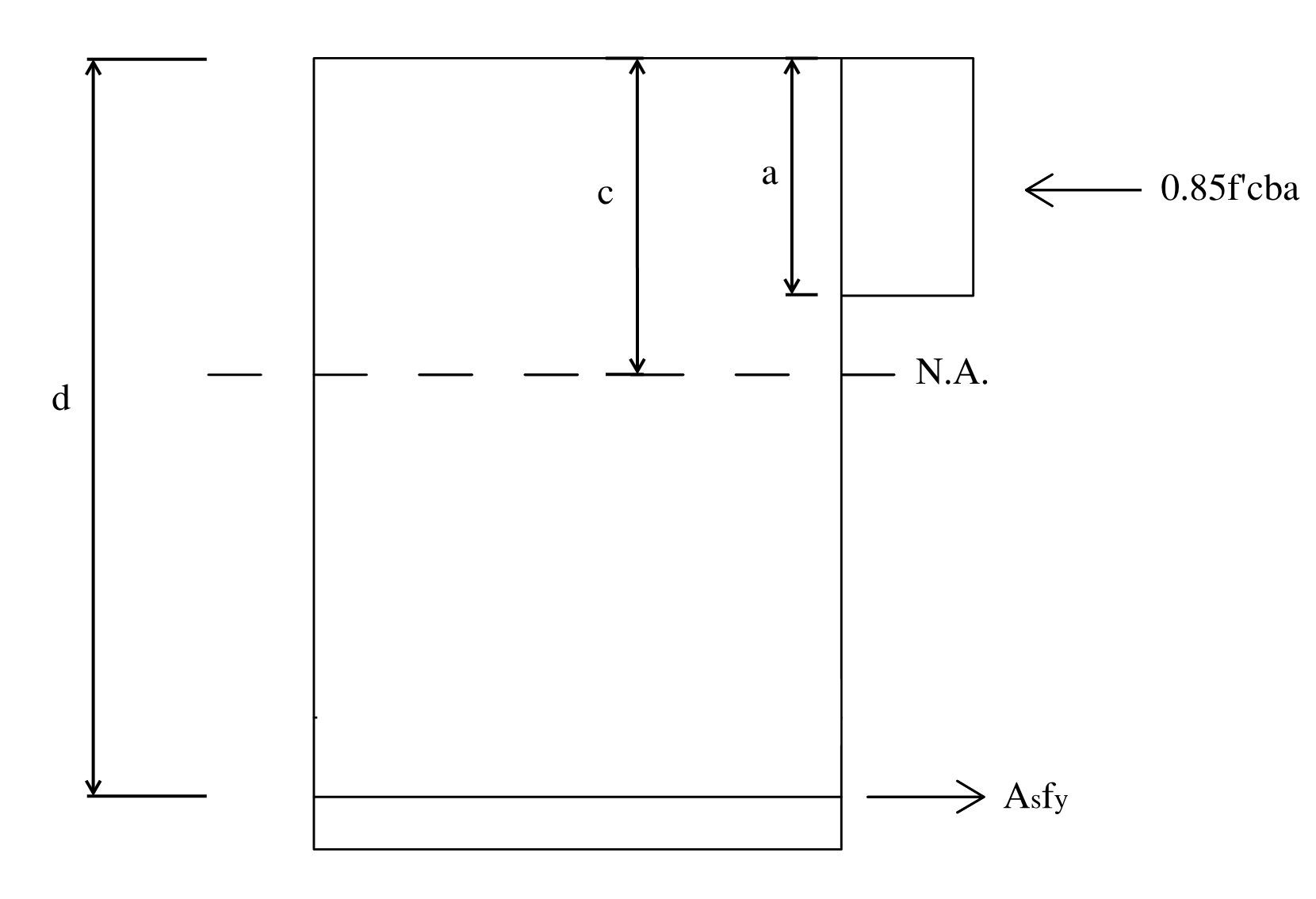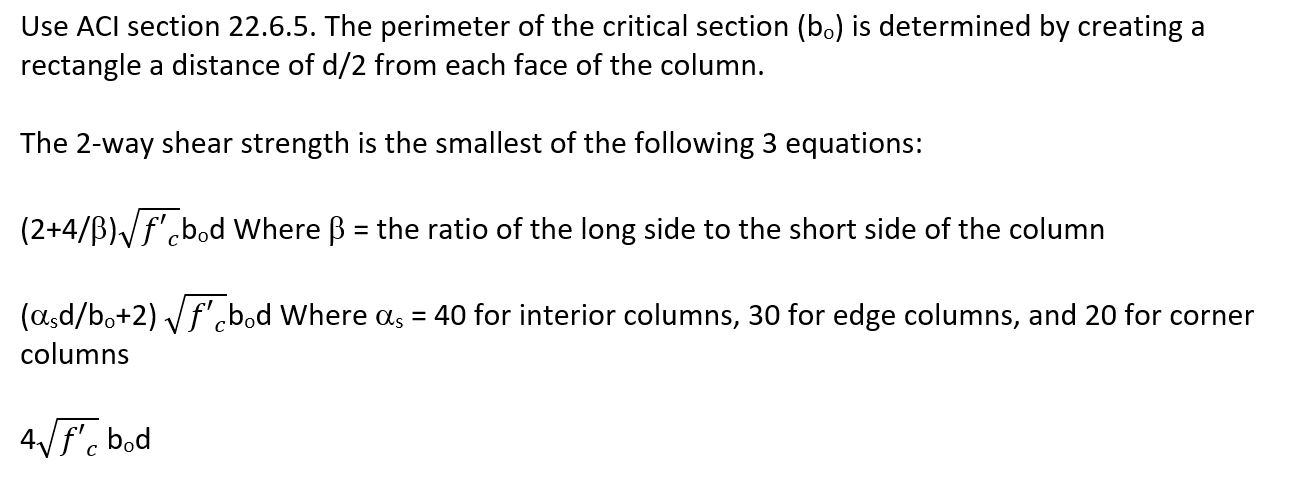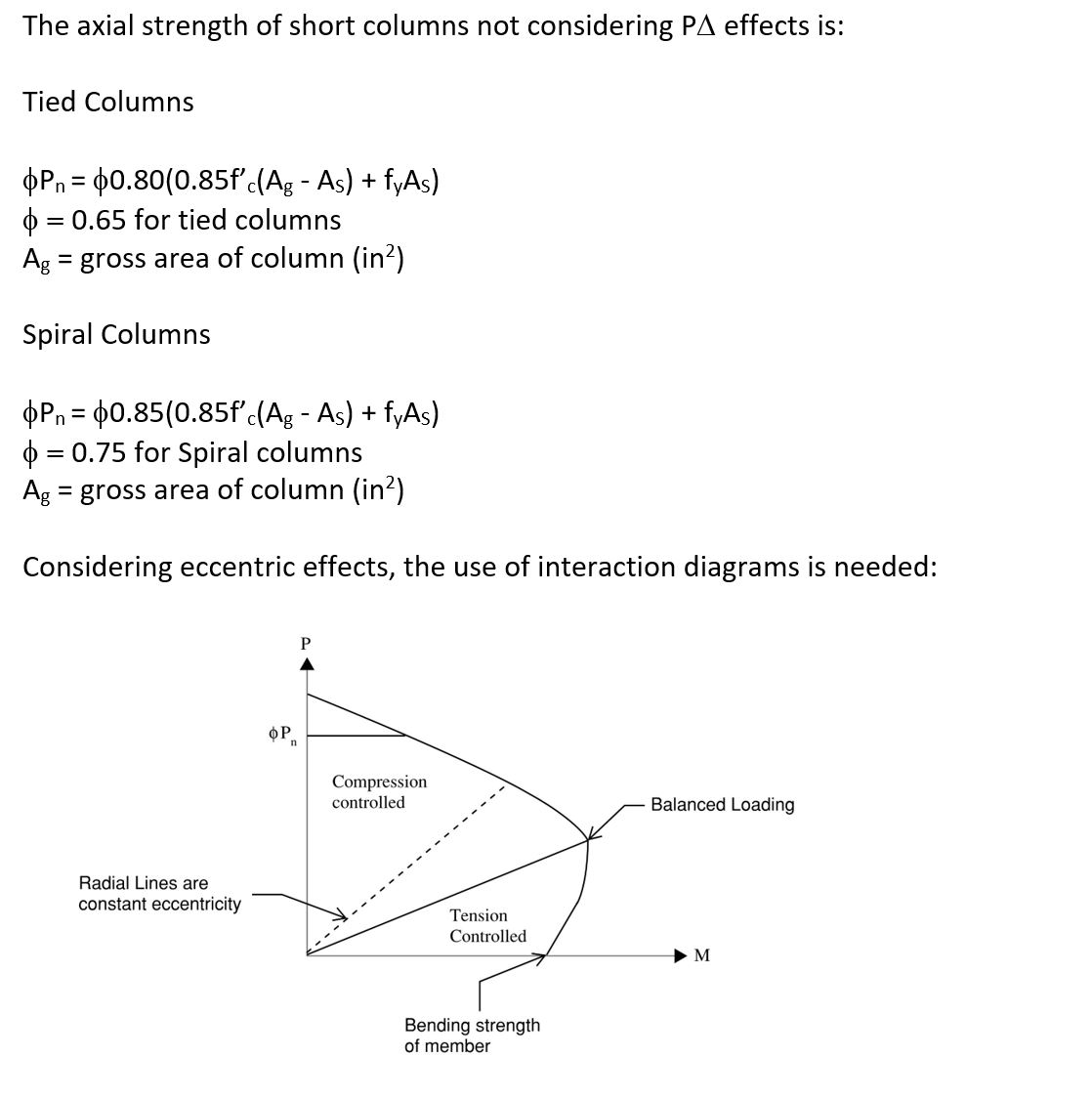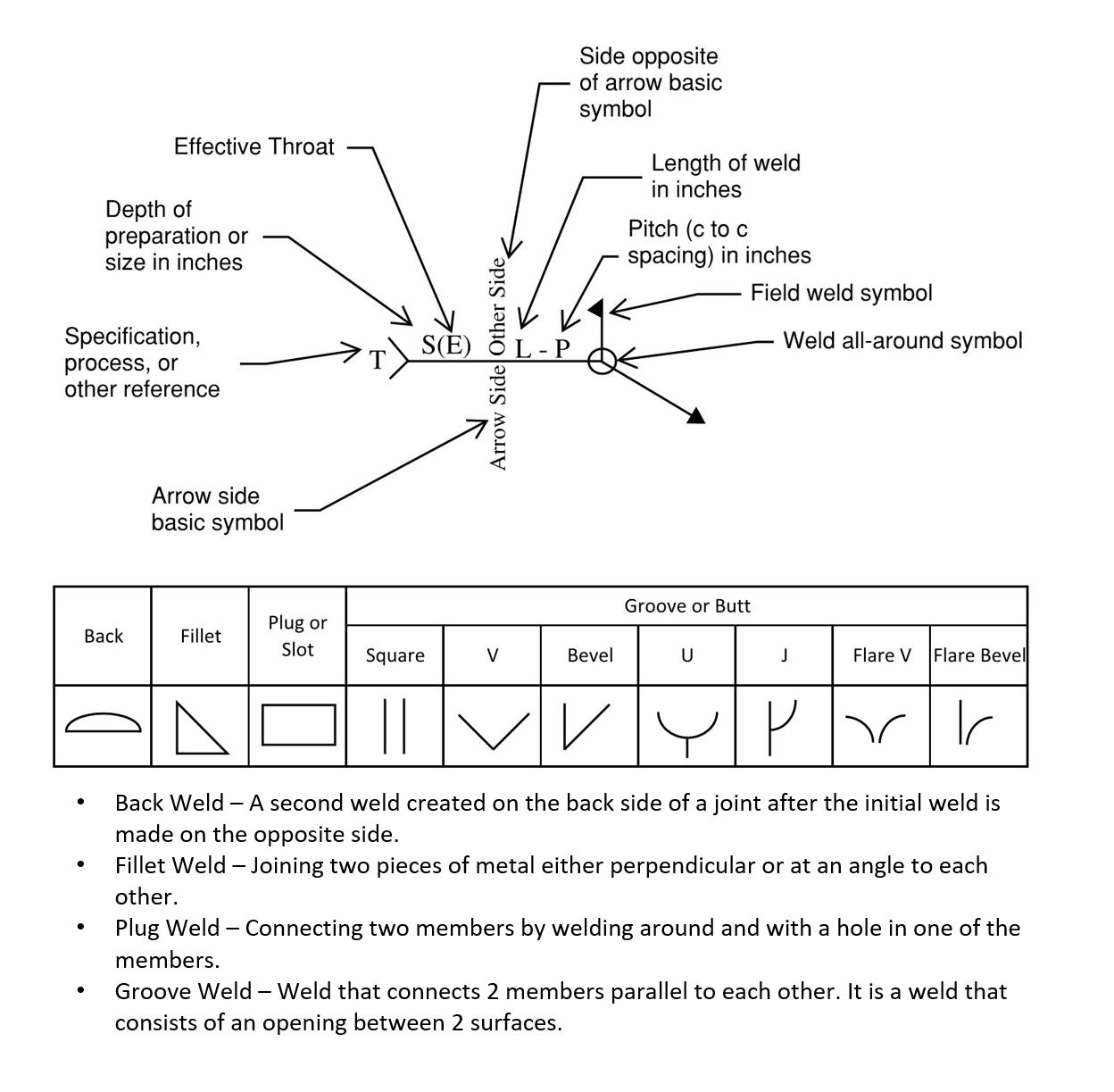## PE Exam - Structural Depth

• PE Core Concepts PE Structural Exam Review & Quick Reference Guide designed to break down the specific information needed for the exam on every topic from the NCEES Syllabus

• Comprehensive PE Civil Engineering Structural Practice Exam​.

• 40 Civil Breadth practice problems with detailed solutions

• 80 Structural Depth practice problems with detailed solutions

• Breakdown of all NCEES listed codes including ACI, AISC, IBC, ASCE, Masonry design, NDS, AASHTO, OSHA, and PCI

• Available in Paperback for \$34.95 or access all of our practice questions Online Only for \$24.99

## ASCE

### General Requirements

Some main concepts include:

• Excavation Safety: Except for excavations in rock, anything deeper than 5 ft must be stabilized to prevent cave-in. This may be achieved by providing appropriate earth retention systems or sloping at appropriate rates. This is determined by the depth of excavation, soil type, and other requirements. 1926 Subpart P – Excavations

• Fall protection: Drop-offs must be protected from fall based on the height of the drop. Some examples of protection include temporary fences, nets, or lifelines. 1910 Subpart D – Walking Working Surfaces and 1926 Subpart M – Fall Protection

• Power Line Hazards: For power lines which are electrified, all construction activities must be a minimum distance from the lines. This is based on the voltage of the lines. Typically the safe operational distance is 10 ft. for lines less than 50 kV and typically 35 ft. for lines greater than 50 kV. 1926 Subpart V – Electric Power Transmission and Distribution

• Confined Spaces: Anyone required to enter confined spaces must be appropriately trained and equipped. Oxygen must be monitored and kept at an acceptable level. Subpart AA – Confined Spaces

• Personal Protective Equipment (PPE): Equipment required by any personnel present on a job site. Examples are acceptable head protection and steel toed shoes. 1910 Subpart I – Personal Protective Equipment

## AASHTO

### Flexure

Moment capacity in concrete beams is based on the tension in the member being equal to the compression. The moment capacity then is the area of steel multiplied by the strength of steel multiplied by the distance from the steel centroid to the centroid of the compression block. Therefore:

As = area of steel (in2)

Fy = yield strength of steel (ksi)

d = depth of tension steel (in)

a = depth of compression block (in)

And since Tension = Compression

Asfy = 0.85f’cba, and therefore a = Asfy/(0.85f’cb)

This is represented in the diagram below:The minimum reinforcing in a concrete beam is the larger of the following two equations:The maximum reinforcing does not have a simple equation but is a function of limiting the strain in the steel so that the mode of failure is not crushing of the concrete. This is done by setting the strain of steel to 0.005. Therefore:### Shear

The shear capacity of a concrete beam is the addition of the shear strength of the concrete and the reinforcing stirrups. Therefore:s = spacing of stirrups (in)

Av = Area of vertical stirrups (in2). Note: the cross section for shear often includes multiple vertical bars. Av is the total area of all vertical legs

Spacing shall not be greater than = Avfy/50bw

### Two-Way Shear### AxialACI also provides limits for the reinforcing of members in compression:

• Code Requirements for columns:
• Minimum Longitudinal steel > 0.01Ag
• Maximum Longitudinal steel < 0.08Ag
• Minimum Number of Bars:
• 4 for rectangular ties
• 3 for triangular ties
• 6 for spiral ties
• Minimum size tie is #3 for #10 bars and smaller, #4 for #10 bars and larger
• Center to center tie spacing shall not be greater than:
• 16(longitudinal bar diameter)
• 48(tie diameter)
• Least dimension of the column

### Reinforcing Development and Details## ACI

### Flexure

Moment capacity in concrete beams is based on the tension in the member being equal to the compression. The moment capacity then is the area of steel multiplied by the strength of steel multiplied by the distance from the steel centroid to the centroid of the compression block. Therefore:

As = area of steel (in2)

Fy = yield strength of steel (ksi)

d = depth of tension steel (in)

a = depth of compression block (in)

And since Tension = Compression

Asfy = 0.85f’cba, and therefore a = Asfy/(0.85f’cb)

This is represented in the diagram below:The minimum reinforcing in a concrete beam is the larger of the following two equations:The maximum reinforcing does not have a simple equation but is a function of limiting the strain in the steel so that the mode of failure is not crushing of the concrete. This is done by setting the strain of steel to 0.005. Therefore:### Shear

The shear capacity of a concrete beam is the addition of the shear strength of the concrete and the reinforcing stirrups. Therefore:s = spacing of stirrups (in)

Av = Area of vertical stirrups (in2). Note: the cross section for shear often includes multiple vertical bars. Av is the total area of all vertical legs

Spacing shall not be greater than = Avfy/50bw

### Two-Way Shear### AxialACI also provides limits for the reinforcing of members in compression:

• Code Requirements for columns:
• Minimum Longitudinal steel > 0.01Ag
• Maximum Longitudinal steel < 0.08Ag
• Minimum Number of Bars:
• 4 for rectangular ties
• 3 for triangular ties
• 6 for spiral ties
• Minimum size tie is #3 for #10 bars and smaller, #4 for #10 bars and larger
• Center to center tie spacing shall not be greater than:
• 16(longitudinal bar diameter)
• 48(tie diameter)
• Least dimension of the column

### Reinforcing Development and Details## AISC

### Weld Symbols and Types## NDS

The bolt which will see the largest resultant force in a bolt group is the upper most bolt on the side of which the force is appliedVp = vertical force per bolt = P/N

P = applied force (kips)

N = number of bolts

Ve = Force due to eccentricity = Pexc/Io

Xc = distance in X direction from centroid of bolt group to the subject bolt

The moment of inertia the sum of the distances of all bolts in the x and y directions if all bolts have the same diameter. Sum the distances of each bolt to the appropriate neutral axis:The elongation or contraction of a beam due to an applied force is determined from the following equation:

D = PL/AE

P = applied force (kips)

L = length of beam (in)

A = cross sectional area (in2)

E = modulus of elasticity (ksi)

The thermal elongation or contraction for steel is determined by the following equation:

D = Loa(t1 - to)

Lo = original length of beam (in)

a = coefficient of thermal expansion

t1 = new temperature (degrees Fahrenheit)

to = original temperature (degrees Fahrenheit)

### Composite Beams

For composite members, the stresses in the beams can only be determined by analysis of similar materials. To do this, the stronger material should be converted to an equivalent area of the weaker material. This is done using the ratio of the moduli of elasticity n = E1/E2

Then calculate the equivalent width of the weaker material bequiv = bn

The member can then be analyzed as a single material.

### Torsional Shear### Deflections

• Not all deflections are covered under beam chart equations
• Moment Area Method can be used for the determination of angle and deflection
• First draw the moment diagram
• Determine the M/EI diagram by dividing points on the moment diagram by EI
• The angle between tangents of two points is the area of the M/EI diagram between points
• The deflection of a point from the tangent of another is area of the M/EI times the distance from the centroid of this area to the desired point of deflection

### Horizontal Shear Stress

Horizontal shear stress

t = VQ/Ib

V = Applied Shear Force (kips)

Q = First Moment of the Desired Area = ay.

a = Cross Sectional Area from Point of Desired Shear Stress to Extreme Fiber (in2)

y = Distance from Centroid of Beam to Centroid of Area “a” (in)

I = Moment of Inertia of Beam (in3)

b = Width of Member (in)

## ACI 530 Masonry

### General Requirements

Some main concepts include:

• Excavation Safety: Except for excavations in rock, anything deeper than 5 ft must be stabilized to prevent cave-in. This may be achieved by providing appropriate earth retention systems or sloping at appropriate rates. This is determined by the depth of excavation, soil type, and other requirements. 1926 Subpart P – Excavations

• Fall protection: Drop-offs must be protected from fall based on the height of the drop. Some examples of protection include temporary fences, nets, or lifelines. 1910 Subpart D – Walking Working Surfaces and 1926 Subpart M – Fall Protection

• Power Line Hazards: For power lines which are electrified, all construction activities must be a minimum distance from the lines. This is based on the voltage of the lines. Typically the safe operational distance is 10 ft. for lines less than 50 kV and typically 35 ft. for lines greater than 50 kV. 1926 Subpart V – Electric Power Transmission and Distribution

• Confined Spaces: Anyone required to enter confined spaces must be appropriately trained and equipped. Oxygen must be monitored and kept at an acceptable level. Subpart AA – Confined Spaces

• Personal Protective Equipment (PPE): Equipment required by any personnel present on a job site. Examples are acceptable head protection and steel toed shoes. 1910 Subpart I – Personal Protective Equipment

## PCI

Load combinations as per IBC are:

Section 1605.2

• 1.4(D +F) (Equation 16-1)
• 1.2(D + F) + 1.6(L + H) + 0.5(Lr or S or R) (Equation 16-2)
• 1.2(D + F) + 1.6(Lr or S or R) + 1.6H + (f1L or 0.5W) (Equation 16-3)
• 1.2(D + F) + 1.0W + f1L + 1.6H + 0.5(Lr or S or R) (Equation 16-4)
• 1.2(D + F) + 1.0E + f1L + 1.6H + f2S (Equation 16-5)
• 0.9D+ 1.0W+ 1.6H (Equation 16-6)
• 0.9(D + F) + 1.0E+ 1.6H (Equation 16-7)

• f1 = 1 for places of public assembly live loads in excess of 100 pounds per square foot (4.79 kN/m2), and parking garages; and 0.5 for other live loads.
• f2 = 0.7 for roof configurations (such as saw tooth) that do not shed snow off the structure, and 0.2 for other roof configurations.

In addition, the following exceptions apply:

1. Where other factored load combinations are specifically required by other provisions of this code, such combinations shall take precedence.
1. Where the effect of H resists the primary variable load effect, a load factor of 0.9 shall be included with H where H is permanent and H shall be set to zero for all other conditions.

### Special Inspections

• Special Inspections – Inspections of construction materials and components by qualified professionals for compliance with codes and construction documents
• Can be continuous (Full time) or periodic (Part time or intermittent)

## OSHA

### General Requirements

Some main concepts include:

• Excavation Safety: Except for excavations in rock, anything deeper than 5 ft must be stabilized to prevent cave-in. This may be achieved by providing appropriate earth retention systems or sloping at appropriate rates. This is determined by the depth of excavation, soil type, and other requirements. 1926 Subpart P – Excavations

• Fall protection: Drop-offs must be protected from fall based on the height of the drop. Some examples of protection include temporary fences, nets, or lifelines. 1910 Subpart D – Walking Working Surfaces and 1926 Subpart M – Fall Protection

• Power Line Hazards: For power lines which are electrified, all construction activities must be a minimum distance from the lines. This is based on the voltage of the lines. Typically the safe operational distance is 10 ft. for lines less than 50 kV and typically 35 ft. for lines greater than 50 kV. 1926 Subpart V – Electric Power Transmission and Distribution

• Confined Spaces: Anyone required to enter confined spaces must be appropriately trained and equipped. Oxygen must be monitored and kept at an acceptable level. Subpart AA – Confined Spaces

• Personal Protective Equipment (PPE): Equipment required by any personnel present on a job site. Examples are acceptable head protection and steel toed shoes. 1910 Subpart I – Personal Protective Equipment

## IBC

Load combinations as per IBC are:

Section 1605.2

• 1.4(D +F) (Equation 16-1)
• 1.2(D + F) + 1.6(L + H) + 0.5(Lr or S or R) (Equation 16-2)
• 1.2(D + F) + 1.6(Lr or S or R) + 1.6H + (f1L or 0.5W) (Equation 16-3)
• 1.2(D + F) + 1.0W + f1L + 1.6H + 0.5(Lr or S or R) (Equation 16-4)
• 1.2(D + F) + 1.0E + f1L + 1.6H + f2S (Equation 16-5)
• 0.9D+ 1.0W+ 1.6H (Equation 16-6)
• 0.9(D + F) + 1.0E+ 1.6H (Equation 16-7)

• f1 = 1 for places of public assembly live loads in excess of 100 pounds per square foot (4.79 kN/m2), and parking garages; and 0.5 for other live loads.
• f2 = 0.7 for roof configurations (such as saw tooth) that do not shed snow off the structure, and 0.2 for other roof configurations.

In addition, the following exceptions apply:

1. Where other factored load combinations are specifically required by other provisions of this code, such combinations shall take precedence.
1. Where the effect of H resists the primary variable load effect, a load factor of 0.9 shall be included with H where H is permanent and H shall be set to zero for all other conditions.

### Special Inspections

• Special Inspections – Inspections of construction materials and components by qualified professionals for compliance with codes and construction documents
• Can be continuous (Full time) or periodic (Part time or intermittent)

## AWS

### Weld Symbols and TypesLoad combinations as per IBC are:

Section 1605.2

• 1.4(D +F) (Equation 16-1)
• 1.2(D + F) + 1.6(L + H) + 0.5(Lr or S or R) (Equation 16-2)
• 1.2(D + F) + 1.6(Lr or S or R) + 1.6H + (f1L or 0.5W) (Equation 16-3)
• 1.2(D + F) + 1.0W + f1L + 1.6H + 0.5(Lr or S or R) (Equation 16-4)
• 1.2(D + F) + 1.0E + f1L + 1.6H + f2S (Equation 16-5)
• 0.9D+ 1.0W+ 1.6H (Equation 16-6)
• 0.9(D + F) + 1.0E+ 1.6H (Equation 16-7)

• f1 = 1 for places of public assembly live loads in excess of 100 pounds per square foot (4.79 kN/m2), and parking garages; and 0.5 for other live loads.
• f2 = 0.7 for roof configurations (such as saw tooth) that do not shed snow off the structure, and 0.2 for other roof configurations.

In addition, the following exceptions apply:

1. Where other factored load combinations are specifically required by other provisions of this code, such combinations shall take precedence.
1. Where the effect of H resists the primary variable load effect, a load factor of 0.9 shall be included with H where H is permanent and H shall be set to zero for all other conditions.

### Special Inspections

• Special Inspections – Inspections of construction materials and components by qualified professionals for compliance with codes and construction documents
• Can be continuous (Full time) or periodic (Part time or intermittent)

## Misc. Structural Topics

The bolt which will see the largest resultant force in a bolt group is the upper most bolt on the side of which the force is appliedVp = vertical force per bolt = P/N

P = applied force (kips)

N = number of bolts

Ve = Force due to eccentricity = Pexc/Io

Xc = distance in X direction from centroid of bolt group to the subject bolt

The moment of inertia the sum of the distances of all bolts in the x and y directions if all bolts have the same diameter. Sum the distances of each bolt to the appropriate neutral axis:The elongation or contraction of a beam due to an applied force is determined from the following equation:

D = PL/AE

P = applied force (kips)

L = length of beam (in)

A = cross sectional area (in2)

E = modulus of elasticity (ksi)

The thermal elongation or contraction for steel is determined by the following equation:

D = Loa(t1 - to)

Lo = original length of beam (in)

a = coefficient of thermal expansion

t1 = new temperature (degrees Fahrenheit)

to = original temperature (degrees Fahrenheit)

### Composite Beams

For composite members, the stresses in the beams can only be determined by analysis of similar materials. To do this, the stronger material should be converted to an equivalent area of the weaker material. This is done using the ratio of the moduli of elasticity n = E1/E2

Then calculate the equivalent width of the weaker material bequiv = bn

The member can then be analyzed as a single material.

### Torsional Shear### Deflections

• Not all deflections are covered under beam chart equations
• Moment Area Method can be used for the determination of angle and deflection
• First draw the moment diagram
• Determine the M/EI diagram by dividing points on the moment diagram by EI
• The angle between tangents of two points is the area of the M/EI diagram between points
• The deflection of a point from the tangent of another is area of the M/EI times the distance from the centroid of this area to the desired point of deflection

### Horizontal Shear Stress

Horizontal shear stress

t = VQ/Ib

V = Applied Shear Force (kips)

Q = First Moment of the Desired Area = ay.

a = Cross Sectional Area from Point of Desired Shear Stress to Extreme Fiber (in2)

y = Distance from Centroid of Beam to Centroid of Area “a” (in)

I = Moment of Inertia of Beam (in3)

b = Width of Member (in)

###### Civil PE Exam Structural Depth Sample Practice Problems